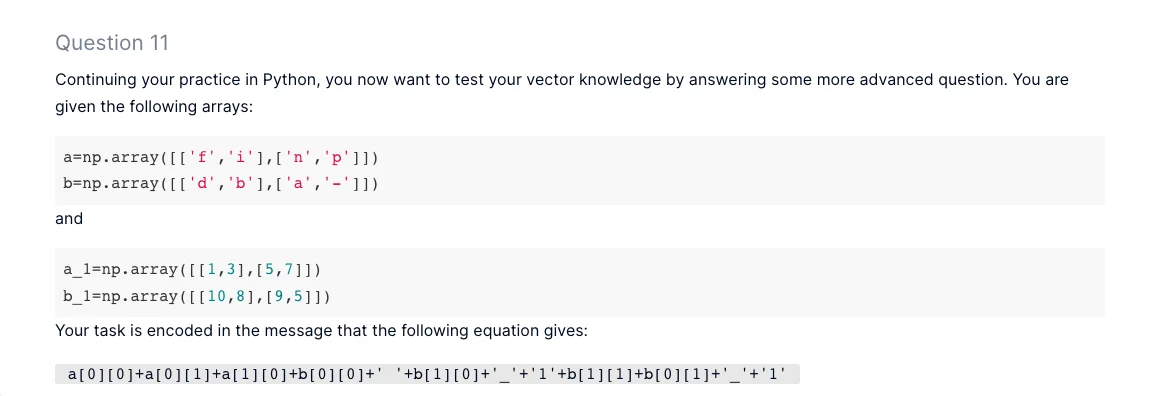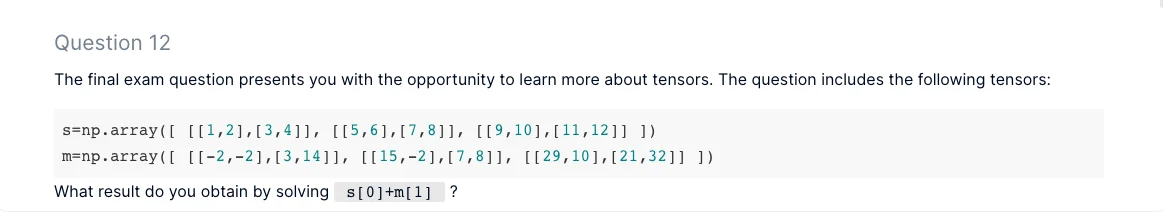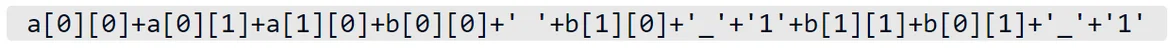15 Jun 2022

Posted on:

13 Jun 2022

0

# not understanding two questions for the mathematics course exam

I don't understand how i am supposed to interpret the encoded equation from the arrays given. The answer was to find a_1-b_1. i don't understand how that conclusion was drawn from encoded equation. I would like clarification on that.The operation for question 12 ( s+m ) i didn't initially understand. but then i remembered briefly in the mathematic lesson that in python that the index loop always starts at 0 instead of 1 like in general math. Is that correct? i would like that clarified.Instructor
Posted on:

15 Jun 2022

1

Hey George,

Regarding Question 11 from the exam, the task is encoded in the expressionYou are given the matrices `a`, `b`, `a_1`, and `b_1` above. The task is, therefore, to find the following symbols in the matrices and formulate the question.

``````a
a
a
b
b
b
b
``````

The plus signs concatenate the respective strings. Once you've found what the task is, you need to perform it.

Regarding Question 12 from the same exam, `s` is indeed the first item while `m` is the second. Once you locate them, you would need to find their sum.

Hope this clarifies the questions!

Kind regards,
365 Hristina